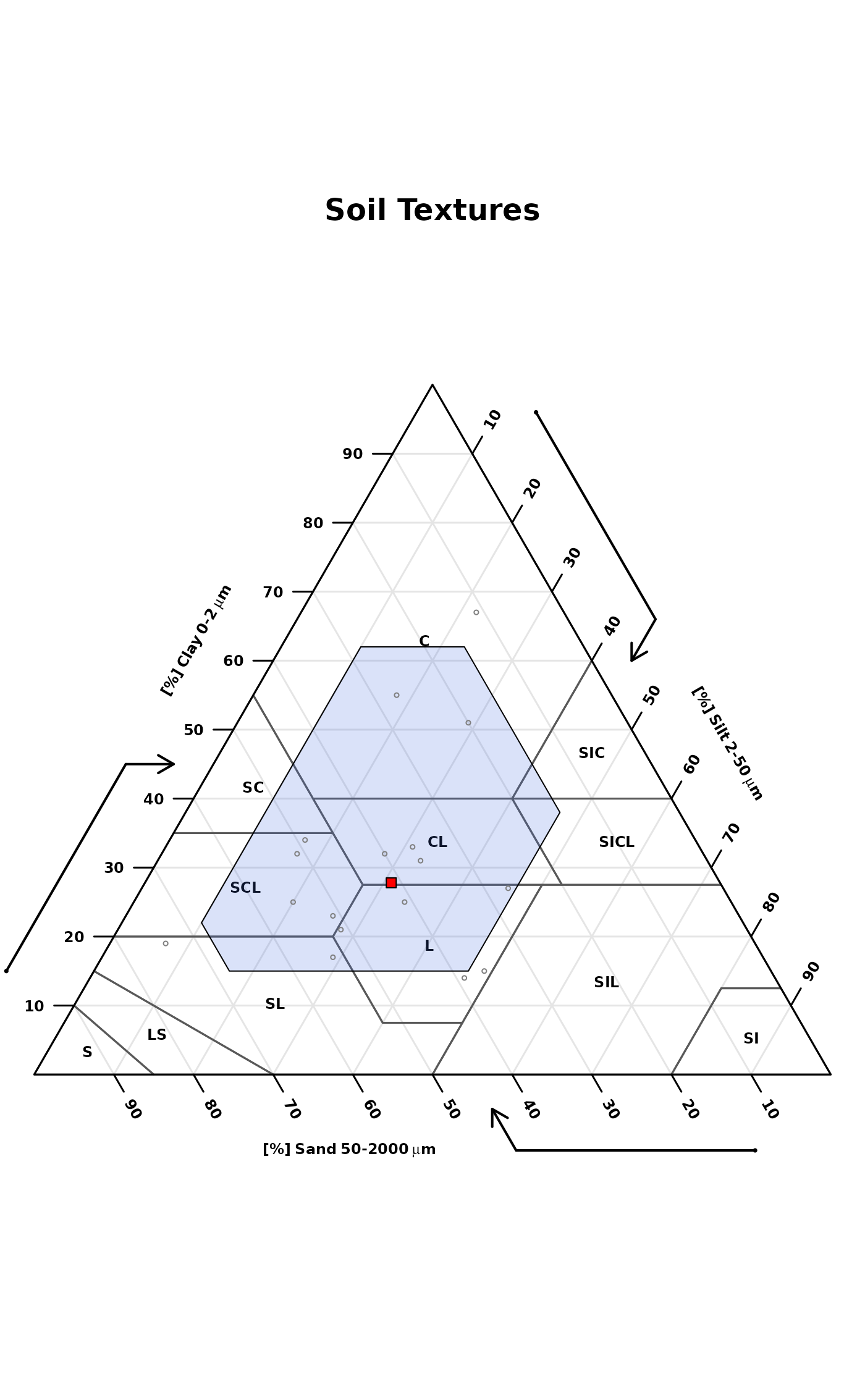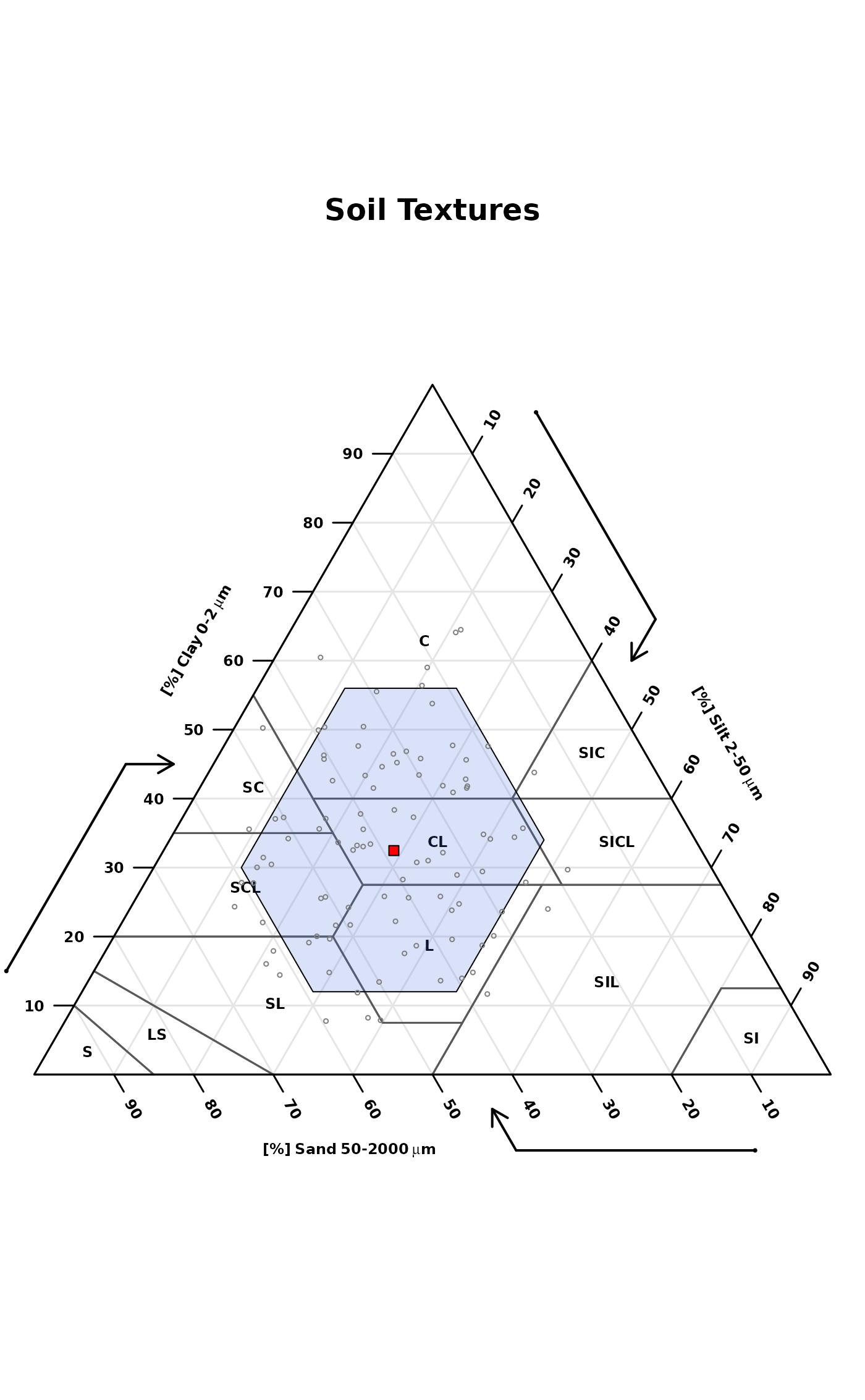This function accepts soil texture components (sand, silt, and clay percentages) and plots a soil texture triangle with a "representative value" (point) and low-high region (polygon) defined by quantiles (estimated with Hmisc::hdquantile). Marginal quantiles of sand, silt, and clay are used to define the boundary of a low-high region. The default settings place the RV symbol at the texture defined by marginal medians of sand, silt, and clay. The default low-high region is defined by the 5th and 95th marginal percentiles of sand, silt, and clay.

textureTriangleSummary(
ssc,
p = c(0.05, 0.5, 0.95),
delta = 1,
rv.col = "red",
range.border = "black",
range.col = "RoyalBlue",
range.alpha = 80,
range.lty = 1,
range.lwd = 2,
main = "Soil Textures",
legend.cex = 0.75,
legend = TRUE,
...
)

## Arguments

ssc

data.frame with columns: 'SAND', 'SILT', 'CLAY', values are percentages that should add to 100. No NA allowed.

p

vector of percentiles (length = 3) defining 'low', 'representative value', and 'high'

delta

grid size used to form low-high region

rv.col

color used for representative value (RV) symbol

range.border

color used for polygon border enclosing the low-high region

range.col

color used for polygon enclosing the low-high region

range.alpha

transparency of the low-high range polygon (0-255)

range.lty

line style for polygon enclosing the low-high region

range.lwd

line weight polygon enclosing the low-high region

main

plot title

legend.cex

scaling factor for legend

legend

logical, enable/disable automatic legend

...

further arguments passed to soiltexture::TT.points

## Value

an invisible matrix with marginal percentiles of sand, silt, and clay

bootstrapSoilTexture

## Author

D.E. Beaudette, J. Nemecek, K. Godsey

## Examples


# \donttest{

if(
requireNamespace("Hmisc") &
requireNamespace("compositions") &
requireNamespace("soiltexture")
) {

# sample data
data('sp4')

# subset rows / columns
ssc <- sp4[grep('^Bt', sp4$name), c('sand', 'silt', 'clay')] names(ssc) <- toupper(names(ssc)) # make figure, marginal percentiles are silently returned stats <- textureTriangleSummary( ssc, pch = 1, cex = 0.5, range.alpha = 50, range.lwd = 1, col = grey(0.5), legend = FALSE ) # check stats # simulate some data and try again s <- bootstrapSoilTexture(ssc, n = 100)$samples

# make the figure, ignore results
textureTriangleSummary(
s, pch = 1, cex = 0.5,
range.alpha = 50,
range.lwd = 1,
col = grey(0.5),
legend = FALSE
)
}# }